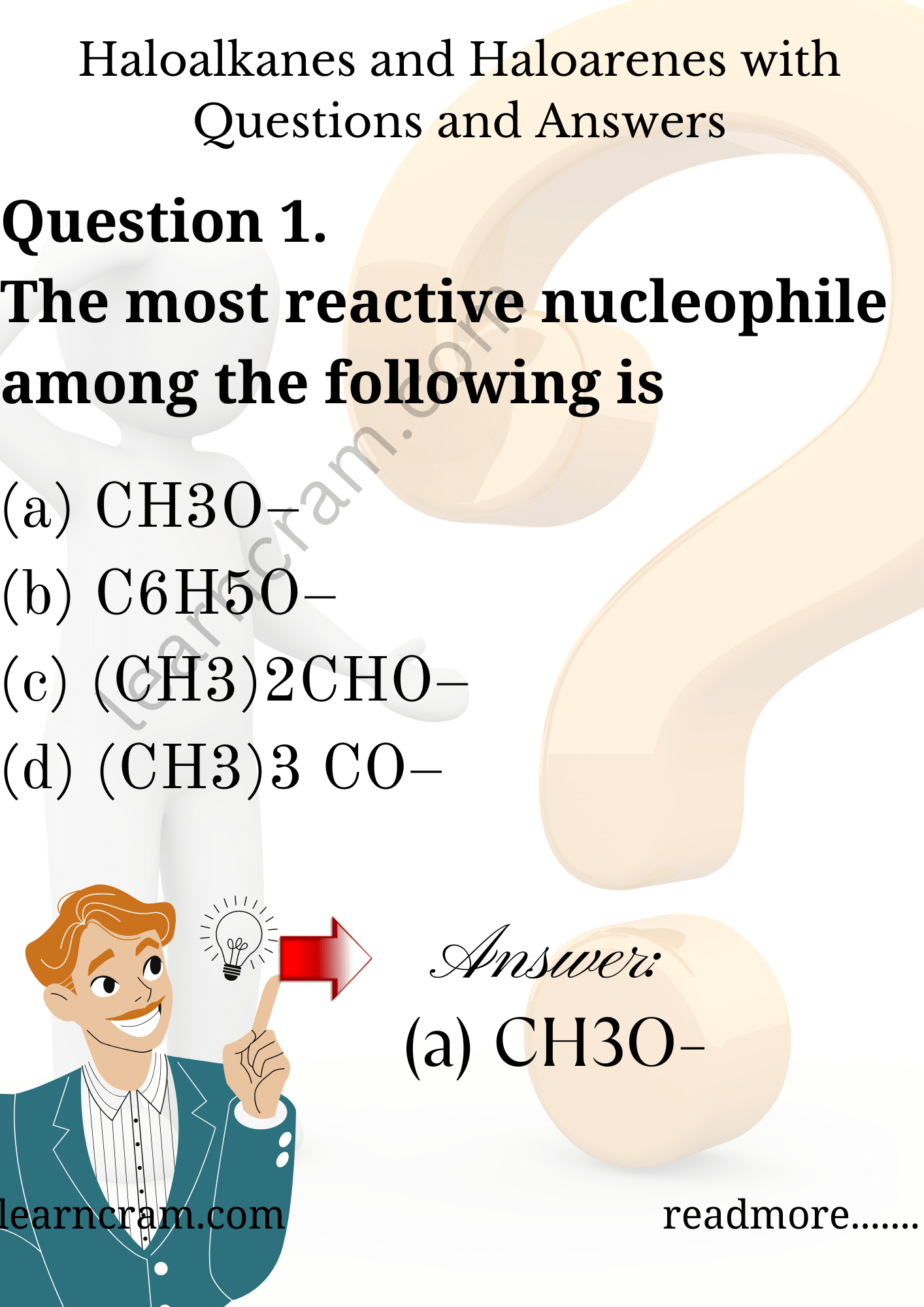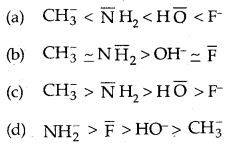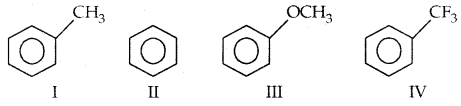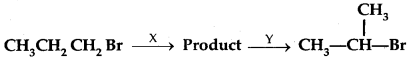# MCQ Questions for Class 12 Chemistry Chapter 10 Haloalkanes and Haloarenes with Answers

We have compiled the NCERT MCQ Questions for Class 12 Chemistry Chapter 10 Haloalkanes and Haloarenes with Answers Pdf free download covering the entire syllabus. Practice MCQ Questions for Class 12 Chemistry with Answers on a daily basis and score well in exams. Refer to the Haloalkanes and Haloarenes Class 12 MCQs Questions with Answers here along with a detailed explanation.

## Haloalkanes and Haloarenes Class 12 MCQs Questions with Answers

Question 1.
The most reactive nucleophile among the following is
(a) CH3O
(b) C6H5O
(c) (CH3)2CHO
(d) (CH3)3 COQuestion 2.
CH3CH2CHCl CH3 obtained by chlorination of n-butane, will be
(a) meso-form
(b) racemic mixture
(c) d-form
(d) 1-formQuestion 3.
In Friedel-Crafts synthesis of toluene, reactants in addition to anhydrous AlCl3 are:
(a) C6H6 + CH4
(b) C6H6 + CH3Cl
(c) C5H5Cl + CH3Cl
(d) C6H5/sub>Cl + CH4

Question 4.
SN1 reaction of alkyl halides leads to
(a) retention of configuration
(b) racemisation
(c) inversion of configuration
(d) none of these.

Question 5.
Nucleophilieity order is correctly represented byQuestion 6.
Which of the following are arranged in the decreasing order of dipole moment?
(a) CH3Cl, CH3Br, CH2F
(b) CH3Cl, CH3F, CH3Br
(c) CH3Br, CH3Cl, CH3F
(d) CH3Br, CH3F, CH3Cl

Question 7.
Which of the following is a free radical substitution reaction?(d) CH3CHO + HCN → CH3CH (OH) CN

Question 8.
The reactivity order of halides for dehydrogenation is
(a) RF > RCl > RBr > RI
(b) RI > RBr > RCl > RF
(c) RI > RCl > RBr > RF
(d) RF > RI > RBr > RCl

Answer: (b) RI > RBr > RCl > RF

Question 9.
The addition of HBr is easiest with
(a) CH2 = CHCl
(b) ClCH = CHCl
(c) CH3-CH = CH2
(d) (CH3)2C = CH2

Question 10.
Among the following compounds, the decreasing order of reactivity towards electrophilic substitution is(a) III > I > II > IV
(b) IV > I > II > III
(c) I > II > III > IV
(d) II > I > III > IV

Answer: (a) III > I > II > IV

Question 11.
Unpleasant smelling carbylamines are formed by heating alkali and chloroform with
(a) Any aliphatic amine
(b) Any aromatic amine
(c) Any amine
(d) Any primary amine

Question 12.
Which among MeX, R-CH2X, R2CHX, R3CX is most reactive towards SN2 reaction
(a) MeX
(b) RCH2X
(c) R3CHX
(d) R3CX

Question 13.
Number of stereoisomers of the compound 2-chloro-4- methylhex-2-ene is /are
(a) 1
(b) 2
(c) 4
(d) 16

Question 14.
In chlorination of benzene, the reactive species is
(a) Cl+
(b) Cl
(c) Cl2
(d) Cl$$_{2}^{-}$$

Question 15.
An organic compound, on treatment with Br2 in CCl4 gives bromo derivative of an alkene. The compound will be
(a) CH4-CH = CH2
(b) CH3-CH = CH-CH3
(c) HC = CH
(d) H2C = CH2

Question 16.
If one H is replaced by a Cl atom in C4H9Cl, the total number of structural isomers will be
(a) 4
(b) 5
(c) 7
(d) 10

Question 17.
Anti-Markovnikov addition of HBr is not observed in
(a) Propene
(b) 1-Butene
(c) But-2-ene
(d) Pent-2-ene

Question 18.
Among the following, the molecule with the highest dipole moment is
(a) CH3Cl
(b) CH2Cl2
(c) CHCl3
(d) CCl4

Question 19.
The number of isomers for the compound with molecular formula C2 Br Cl FI is
(a) 3
(b) 4
(c) 5
(d) 6

Question 20.
(CH3)3 C MgBr on reaction with D2O produces
(a) (CH3)3CD
(b) (CH3)3COD
(c) (CH3)3CD
(d) (CD3)3OD

Question 21.
A solution (+) -2-chloro-2-phenylethane in toluene racemises slowly in the presence of small amount of SbCl5, due to the formation of
(a) Carbanion
(b) Carbene
(d) Carbocation

Question 22.
In the addition of HBr to propene in the absence of peroxides, the fist step involves the addition of
(a) H+
(b) Br
(c) H°
(d) Br°

Question 23.
In the reaction of p-Chlorotoluene with KNH2 in liq. NHy the major product is
(a) o-toluidine
(b) m-toluidine
(c) p-toluidine
(d) p-chloro aniline

Question 24.
During debromination of meso-dibromo butane, the major compound formed is
(a) n-butane
(b) 1-butene
(c) cis-2-butene
(d) trans-2-butene.

Question 25.
The number of possible enantiomeric pairs that can be produced during monochlorination of 2-methyl butane is
(a) 2
(b) 3
(c) 4
(d) 1

Question 26.
Arrange the following compounds in order of increasing dipole moment: Toluene (I), m-dichlorobenzene (II), o- dichlorobenzene (III) and p-dichlorobenzene (IV).
(a) I < IV < II < III
(b) IV < r < II < III
(c) IV < I < III < II
(d) IV < II < I < III

Answer: (b) IV < r < II < III

Question 27.
In the following groups : – O Ac (I), – O Me (II), – O SO2 Me (III), – OSO2 CF3 (IV), The order of leaving group ability is
(a) I > II > III > IV
(b) IV > III > I > II
(c) m > II > I > IV
(d) II > III > IV > I

Answer: (b) IV > III > I > II

Question 28.
Identify the set of reagent/reaction conditions X and Y in the following set of transformations:(a) X = dil. aqueous NaOH, 20° C, Y = HBr/Acetic acid, 20°C
(b) X = Cone, alcoholic NaOH, 80°C, Y = HBr/acetic acid, 20°C
(c) X = dil. aqueous NaOH, 20°C Y – Br2/CHCl3,0°C
(d) X = Cone, alcoholic NaOH, 80°C, Y = Br2/CHCl3, 0°C.

Answer: (b) X = Cone, alcoholic NaOH, 80°C, Y = HBr/acetic acid, 20°C

Question 29.
In the presence of peroxide, hydrogen chloride and hydrogen iodide do not give anti-Markovnikov’s addition to alkenes because
(a) both are highly ionic
(b) one is oxidizing and the other is reducing
(c) one of the steps is endothermic in both the cases
(d) all the steps are exothermic in both the reactions

Answer: (c) one of the steps is endothermic in both the cases

Question 30.
When chlorine is passed through propene at 400°C, which of the following is formed?
(a) FVC
(b) Allyl Chloride
(c) Vinyl Chloride
(d) 1,2-Dichloroethane